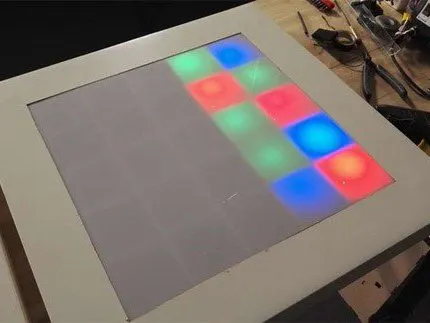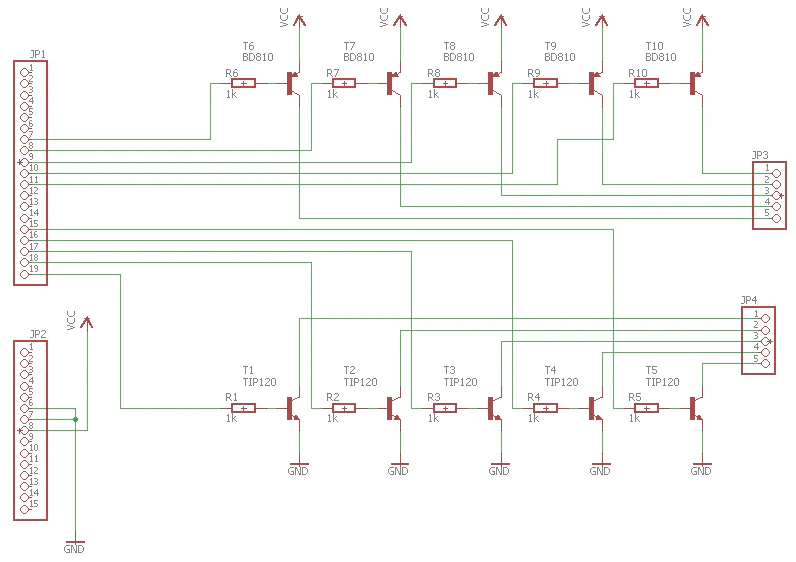# LED Table

Our LED Table is a simple DIY project for Arduino beginners.

BeginnerWork in progress367## Schematics

### schaltplan2_6CsbWQqFj3.png## Code

### Untitled file

Arduino
Let's take a closer look at the outputs of the Arduino and the shield we've attached. The output pins 0 to 4 (5 in total) are connected to transistors that act as switches. If they are supplied with a low current, switch
this. With a HIGH = 1 at the Arduino output, the connected transistor is turned on and all 5 LEDs in the corresponding row are supplied with (+) voltage.
However, the LEDs do not light up. So far, only one strand is "connected" through the transistor. The second is missing, so that electrons can flow away again. If outputs 8 - 12 are set to LOW = 0, the columns will be "connected" to (-) Ground. Again, transistors are used as switches.
As we have seen, the pins of the Arduino act as outputs and are executed with the command pinMode (0, OUTPUT); as such. In addition, the designation "row_" or "column_" is used instead of the pin number of the hardware. This makes it easier to respond to an accurate LED. There is a pin associated with the respective (integer variable) name. With LEDs of different colors, it helps to enumerate the colors [red: R, blue: B, green: G] in the comment in the present sequence.
```int reihe_1
= 0;  //R B R G B
int reihe_2 = 1;  //G G B R G
int reihe_3 = 2;  //B R B G R
int reihe_4 = 3;  //R G R G B
int reihe_5 = 4;  //G B G B R

int spalte_1 = 8;
int spalte_2 = 9;
int spalte_3 = 10;
int spalte_4 = 11;
int spalte_5 = 12;
void setup() {
// put your setup code here, to run
once:
pinMode(reihe_1, OUTPUT);
pinMode(reihe_2, OUTPUT);
pinMode(reihe_3, OUTPUT);
pinMode(reihe_4, OUTPUT);
pinMode(reihe_5, OUTPUT);
pinMode(spalte_1, OUTPUT);
pinMode(spalte_2, OUTPUT);
pinMode(spalte_3, OUTPUT);
pinMode(spalte_4, OUTPUT);
pinMode(spalte_5, OUTPUT);
}
void loop() {
// put your main code here, to run
repeatedly:
for (int s =8; s<=12; s++){
for (int r =0; r<=4; r++){
einzel_LED(r,s);
delay(3000);
}}

}
void
einzel_LED (int reihe, int spalte) {
digitalWrite(reihe_1, LOW);
digitalWrite(reihe_2, LOW);
digitalWrite(reihe_3, LOW);
digitalWrite(reihe_4, LOW);
digitalWrite(reihe_5, LOW);
digitalWrite(spalte_1, LOW);
digitalWrite(spalte_2, LOW);
digitalWrite(spalte_3, LOW);
digitalWrite(spalte_4, LOW);
digitalWrite(spalte_5, LOW);

// Setzen
digitalWrite(reihe, HIGH);
if (not(spalte == 8))
digitalWrite(spalte_1, HIGH);
if (not(spalte == 9))
digitalWrite(spalte_2, HIGH);
if (not(spalte == 10))
digitalWrite(spalte_3, HIGH);
if (not(spalte == 11))
digitalWrite(spalte_4, HIGH);
if (not(spalte == 12))
digitalWrite(spalte_5, HIGH);
}

// In the following source code, individual LEDs in the
turned on and turned off after 3 seconds. The LEDs are looped
switched on one after the other. Before the next switch-on, all LEDs will be lit.
briefly turned off.

int reihe_1 =
0;  //R B R G B
int reihe_2 = 1;  //G G B R G
int reihe_3 = 2;  //B R B G R
int reihe_4 = 3;  //R G R G B
int reihe_5 = 4;  //G B G B R

int spalte_1 = 8;
int spalte_2 = 9;
int spalte_3 = 10;
int spalte_4 = 11;
int spalte_5 = 12;
void setup() {
// put your setup code here, to run
once:
pinMode(reihe_1, OUTPUT);
pinMode(reihe_2, OUTPUT);
pinMode(reihe_3, OUTPUT);
pinMode(reihe_4, OUTPUT);
pinMode(reihe_5, OUTPUT);
pinMode(spalte_1, OUTPUT);
pinMode(spalte_2, OUTPUT);
pinMode(spalte_3, OUTPUT);
pinMode(spalte_4, OUTPUT);
pinMode(spalte_5, OUTPUT);
}
void loop() {
digitalWrite(reihe_1, HIGH);
digitalWrite(reihe_2, HIGH);
digitalWrite(reihe_3, HIGH);
digitalWrite(reihe_4, HIGH);
digitalWrite(reihe_5, HIGH);
digitalWrite(spalte_1, HIGH);
digitalWrite(spalte_2, HIGH);
digitalWrite(spalte_3, HIGH);
digitalWrite(spalte_4, HIGH);
digitalWrite(spalte_5, HIGH);

delay(3000);

digitalWrite(reihe_1, LOW);
digitalWrite(reihe_2, LOW);
digitalWrite(reihe_3, LOW);
digitalWrite(reihe_4, LOW);
digitalWrite(reihe_5, LOW);
digitalWrite(spalte_1, LOW);
digitalWrite(spalte_2, LOW);
digitalWrite(spalte_3, LOW);
digitalWrite(spalte_4, LOW);
digitalWrite(spalte_5, LOW);

delay(3000);
}
```

## Credits

### Makerfactory

6 projects • 6 followers
Practice makes perfect.We are designing the future together with our customers.